Work, Energy, and Power - Lesson 2 - The Work-Energy Relationship

# Internal vs. External Forces

There are a variety of ways to categorize all the types of forces. In a previous unit, it was mentioned that all the types of forces could be categorized as contact forces or as action-at-a-distance forces. Whether a force was categorized as an action-at-a-distance force was dependent upon whether or not that type of force could exist even when the objects were not physically touching. The force of gravity, electrical forces, and magnetic forces were examples of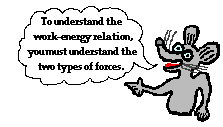forces that could exist between two objects even when they are not physically touching. In this lesson, we will learn how to categorize forces based upon whether or not their presence is capable of changing an object's total mechanical energy. We will learn that there are certain types of forces, that when present and when involved in doing work on objects will change the total mechanical energy of the object. And there are other types of forces that can never change the total mechanical energy of an object, but rather can only transform the energy of an object from potential energy to kinetic energy (or vice versa). The two categories of forces are referred to as internal forces and external forces.

### What Does Work Do?

Forces can be categorized as internal forces or external forces. There are many sophisticated and worthy ways of explaining and distinguishing between internal and external forces. Many of these ways are commonly discussed at great length in physics textbooks - particularly college-level physics textbooks. For our purposes, we will simply say that external forces include the applied force, normal force, tension force, friction force, and air resistance force. And for our purposes, the internal forces include the gravity forces, magnetic force, electrical force, and spring force. While this is a simplistic approach, it is an approach that will serve us well in our introduction to physics.

 Internal Forces External Forces Fgrav Fspring Fapp Ffrict Fair Ftens Fnorm

The importance of categorizing a force as being either internal or external is related to the ability of that type of force to change an object's total mechanical energy when it does work upon an object. When net work is done upon an object by an external force, the total mechanical energy (KE + PE) ofthat object is changed. If the work is positive work, then the object will gain energy. If the work is negative work, then the object will lose energy. The gain or loss in energy can be in the form of potential energy, kinetic energy, or both. Under such circumstances, the work that is done will be equal to the change in mechanical energy of the object. This principle will be discussed in great detail later in this lesson. Because external forces are capable of changing the total mechanical energy of an object, they are sometimes referred to as nonconservative forces.

When the only type of force doing net work upon an object is an internal force (for example, gravitational and spring forces), the total mechanical energy (KE + PE) of that object remains constant. In such cases, the object's energy changes form. For example, as an object is "forced" from a high elevation to a lower elevation by gravity, some of the potential energy of that object is transformed into kinetic energy. Yet, the sum of the kinetic and potential energies remains constant. This is referred to as energy conservation and will be discussed in detail later in this lesson. When the only forces doing work are internal forces, energy changes forms - from kinetic to potential (or vice versa); yet the total amount of mechanical is conserved. Because internal forces are capable of changing the form of energy without changing the total amount of mechanical energy, they are sometimes referred to as conservative forces.In the following descriptions, the only forces doing work upon the objects are internal forces - gravitational and spring forces. Thus, energy is transformed from KE to PE (or vice versa) while the total amount of mechanical energy is conserved. Read each description and indicate whether energy is transformed from KE to PE or from PE to KE. Click the mouse to check your answers.

 Description of Motion KE to PE or PE to KE? Explain. A ball falls from a height of 2 meters in the absence of air resistance.See AnswerPE to KE The ball is losing height (falling) and gaining speed. Thus, the internal or conservative force (gravity) transforms the energy from PE (height) to KE (speed). A skier glides from location A to location B across a friction free ice.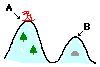See AnswerPE to KE The skier is losing height (the final location is lower than the starting location) and gaining speed (the skier is faster at B than at A). Thus, the internal force or conservative (gravity) transforms the energy from PE (height) to KE (speed). A baseball is traveling upward towards a man in the bleachers.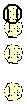See AnswerKE to PE The ball is gaining height (rising) and losing speed (slowing down). Thus, the internal or conservative force (gravity) transforms the energy from KE (speed) to PE (height). A bungee cord begins to exert an upward force upon a falling bungee jumper.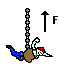See AnswerKE to PE The jumper is losing speed (slowing down) and the bunjee cord is stretching. Thus, the internal or conservative force (spring) transforms the energy from KE (speed) to PE (a stretched "spring"). One might also argue that the gravitational PE is decreasing due to the loss of height. The spring of a dart gun exerts a force on a dart as it is launched from an initial rest position.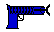See AnswerPE to KE The spring changes from a compressed state to a relaxed state and the dart starts moving. Thus, the internal or conservative force (spring) transforms the energy from PE (a compressed spring) to KE (speed).

NOTE: Perhaps at this time you might find it useful to review the lessons on kinetic energy and potential energy.

### Positive vs. Negative Work and Energy Change

When work is done by external forces (nonconservative forces), the total mechanical energy of the object is altered. The work that is done can be positive work or negative work depending on whether the force doing the work is directed opposite the object's motion or in the same direction as the object's motion. If the force and the displacement are in the same direction, then positive work is done on the object. If positive work is done on an object by an external force, then the object gains mechanical energy. If the force and the displacement are in the opposite direction, then negative work is done on the object; the object subsequently loses mechanical energy.The following descriptions involve external forces (friction, applied, normal, air resistance and tension forces) doing work upon an object. Read the description and indicate whether the object gained energy (positive work) or lost energy (negative work). (NOTE: If this is part is difficult, review the section on work.) Then, indicate whether the gain or loss of energy resulted in a change in the object's kinetic energy, potential energy, or both. Click the buttons to view answers.

Description
+ or - Work?
Change PE or
KE or Both?
Megan drops the ball and hits an awesome forehand. The racket is moving horizontally as the strings apply a horizontal force while in contact with the ball.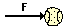A tee ball player hits a long ball off the tee. During the contact time between ball and bat, the bat is moving at a 10 degree angle to the horizontal.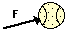Rusty Nales pounds a nail into a block of wood. The hammer head is moving horizontally when it applies force to the nail.The frictional force between highway and tires pushes backwards on the tires of a skidding car.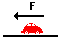A diver experiences a horizontal reaction force exerted by the blocks upon her feet at start of the race.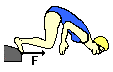A weightlifter applies a force to lift a barbell above his head at constant speed.Note that in the five situations described above, a horizontal force can never change the potential energy of an object. Horizontal forces cannot cause vertical displacements. The only means by which an external or nonconservative force can contribute to a potential energy change is if the force has a vertical component. Potential energy changes are the result of height changes and only a force with a vertical component can cause a height change.### We Would Like to Suggest ...Sometimes it isn't enough to just read about it. You have to interact with it! And that's exactly what you do when you use one of The Physics Classroom's Interactives. We would like to suggest that you combine the reading of this page with the use of our It's All Uphill Interactive and/or our Stopping Distance Interactive. Both Interactives can be found in the Physics Interactive section of our website and provide an interactive opportunity to explore the meaning of the work-energy relationship.

Next Section:

Follow Us